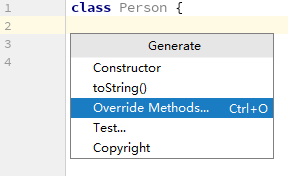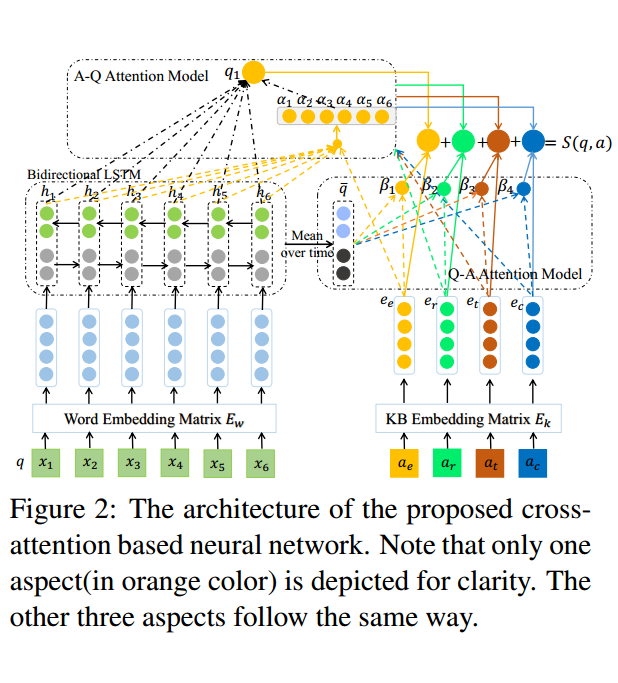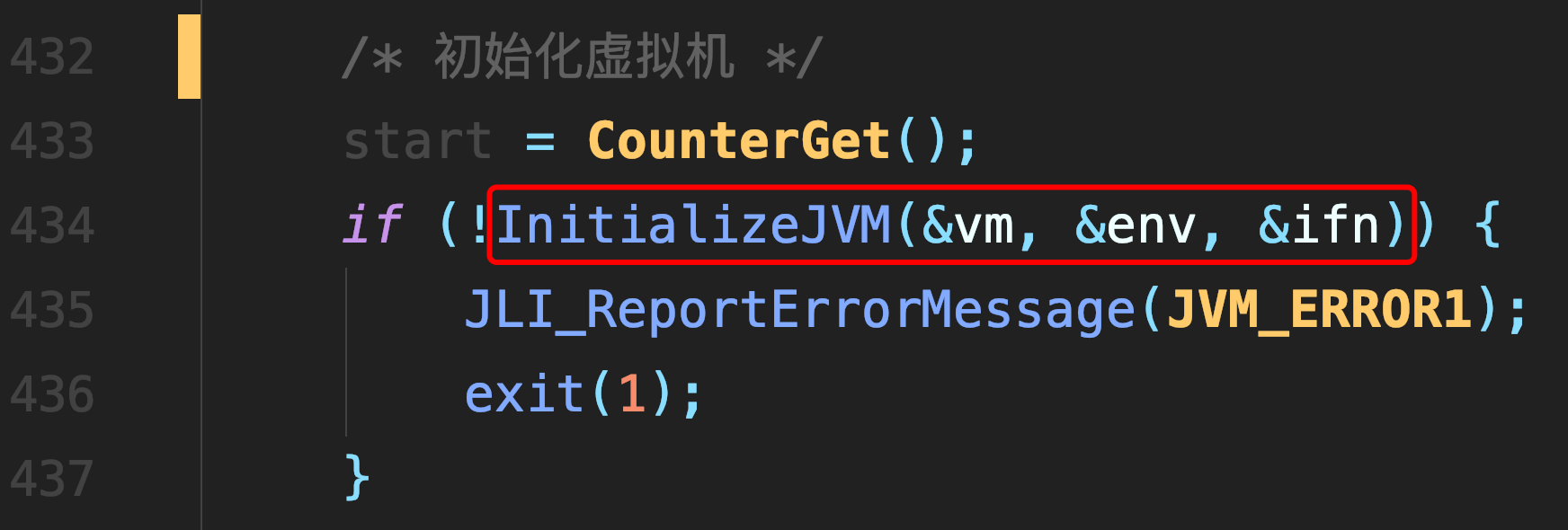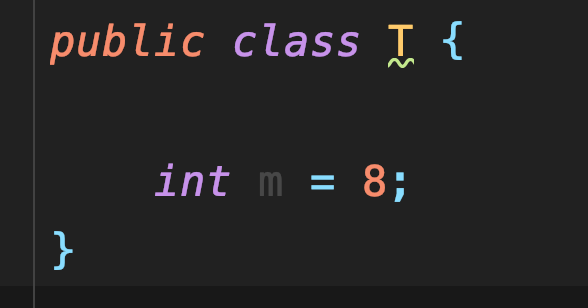# 决策树

### 决策树#### 信息增益

$P(X=x_i)=p_i, i=1,2,....,n$

$H(X)=-\sum\limits_{i=1}^np_i * logp_i$

$H(Y|X)=\sum\limits_{i=1}^np_iH(Y|X=x_i)?$

$p_i=P(X=x_i), i=1,2,...n$

$g(D,A)=H(D)-H(D|A)$

#### ID3算法

ID3算法是最简单的构建决策树算法，具体方法是：从根节点开始，对节点

#### 代码

def createPlot(inTree):
#print (inTree)

fig = plt.figure(1, facecolor='white')
fig.clf()
axprops = dict(xticks=[], yticks=[])
createPlot.ax1 = plt.subplot(111, frameon=False, **axprops)
plotTree.totalW = float(getNumLeafs(inTree))
plotTree.totalD = float(getTreeDepth(inTree))
plotTree.xOff = -0.5/plotTree.totalW
plotTree.yOff = 1.0
plotTree(inTree, (0.5, 1.0), '')
plt.show()


plotTree.totalW是树的叶节点数量

plotTree.totalD是树的深度

plotTree.yOff=1.0容易理解，画布为1.0*1.0,所以初始高度为1.0

plot.xOff=-0.5/plotTree.totalW可能难以理解，不是plot.xOff=0吗？

def plotTree(myTree, parentPt, nodeTxt):
numLeafs = getNumLeafs(myTree)
#depth = getTreeDepth(myTree)
firstStr = list(myTree.keys())
cntrPt = (plotTree.xOff +  1/2 * float(numLeafs)/plotTree.totalW + 0.5/plotTree.totalW, \
plotTree.yOff)
#print (cntrPt)
plotMidText(cntrPt, parentPt, nodeTxt)
plotNode(firstStr, cntrPt, parentPt, decisionNode)
secondDict = myTree[firstStr]
plotTree.yOff = plotTree.yOff - 1.0/plotTree.totalD
for key in secondDict.keys():
if type(secondDict[key]).__name__=='dict':
plotTree(secondDict[key], cntrPt, str(key))
else:
plotTree.xOff = plotTree.xOff + 1.0/plotTree.totalW
plotNode(secondDict[key], (plotTree.xOff, plotTree.yOff),
cntrPt, leafNode)
plotMidText((plotTree.xOff, plotTree.yOff), cntrPt, str(key))
plotTree.yOff = plotTree.yOff + 1.0 / plotTree.totalD


plotTree.xOff + 1/2 * float(numLeafs)/plotTree.totalW + 0.5/plotTree.totalW可能难以理解

##### 热门推荐
•浏览(649)
•浏览(556)
•浏览(540)
•浏览(466)
•浏览(423)
•浏览(402)
•浏览(378)
•浏览(365)
•浏览(355)
•浏览(315)